Here we are providing Class 11 chemistry Important Extra Questions and Answers Chapter 9 Hydrogen. Chemistry Class 11 Important Questions are the best resource for students which helps in Class 11 board exams.

## Class 11 Chemistry Chapter 9 Important Extra Questions Hydrogen

### Hydrogen Important Extra Questions Very Short Answer Type

Question 1.
Which gaseous compound on treatment with dihydrogen produces methanol?
Carbon monoxide (CO).

Question 2.
What are the constituents of water gas?
Carbon monoxide and hydrogen.

Question 3.
Arrange H2, D2, T2 in the decreasing order of their
(i) Boiling point
T2 > D2 > H2

(ii) Heat of fusion.
T2 > D2 > H2.

Question 4.
Which isotope of hydrogen
(i) does not contain neutron
Protium

Tritium.

Question 5.
Out of the following metals which can be used to liberate H2 gas on reaction with dil. hydrochloric acid?
(i) Cu,
(ii) Zn,
(iii) Iron,
(iv) Silver,
(v) Magnesium.
Only Zn, Fe, Mg.

Question 6.
Name one compound each of hydrogen in which it exists in:
(i) Positive oxidation state
HCl

(ii) Negative oxidation state.
NaH.

Question 7.
What is the importance of heavy water in nuclear power generation?
It is used as a moderator in nuclear reactions to slow down the speed of fast-moving neutrons.

Question 8.
State two properties in which hydrogen resembles alkali metals.

1. Both form unipositive ion
2. Both have one electron in their s orbital (ns1).

Question 9.
Give an example of each anionic and covalent hydride.
Ionic Hydride NaH Covalent hydride NH3.

Question 10.
Why is H2O2 concentrated at low pressure?
Because it decomposes at ordinary pressure or on heating.

Question 11.
What is the mass of 1 mole of deuterium oxide and tritium oxide?
D2O = 20g, T2O = 22g.

Question 12.
Arrange H2O, H2S, H2Se, H2Te in the decreasing order of boiling point.
H2O > H2S > H2Se > H2Te.

Question 13.
Give one example of a zeolite used in softening hard water.
Sodium aluminium silicate Na2Al2Si2Og. xH2O.

Question 14.
Name the compounds which retard the decomposition of H2O2.
Acetanilide, glycerol.

Question 15.
Which is heavier out of ice and water?
Water.

Question 16.
What is the trade name of hydrogen peroxide used as an antiseptic?
Perhydrol.

Question 17.
What is the significance of H2O2 labelled as 30 volumes’?
“30 volume” labelled hydrogen peroxide means that 1 mL of this sample of solution gives 30 mL of oxygen gas at STP.

Question 18.
What happens when water is added to calcium carbide.
CaC2 + O → Ca(OH)2 + C2H2
Acetylene gas is produced.

Question 19.
What is the cause of the temporary hardness of water?
Presence of Ca(HCO3)2 and Mg(HCO3)2 in water.

Question 20.
How is the temporary hardness of water removed?
By boiling and filtering.

Question 21.
How is heavy water produced from ordinary water?
By repeated electrolysis of ordinary water containing 3 % of NaOH in it.

Question 22.
Which of the two dihydrogen (H2) or deuterium (D2) undergoes reactions more readily?
Dihydrogen (H2).

Question 23.
Define hard water.
Hard water is one that does not produce lather with soap solution readily.

Question 24.
Which isotope of hydrogen contains an equal number of protons and neutrons?
Deuterium.

Question 25.
What happens when ethylene reacts with hydrogen peroxide?
H2C = CH2 + H2O2 → HO-CH2-CH2-OH
Ethylene glycol

Question 26.
Name the phenomenon of absorption of hydrogen by palladium?
Occlusion.

Question 27.
H2O2 is a better oxidant than H2O. Explain.
H2O2 is easily reduced to form H2O and O.
H2O2 → H2O + O.

Question 28.
Name one example of a reaction in which hydrogen acts as an oxidizing agent.
The reaction of hydrogen with active metals
2Na + H2 → 2NaH .

Question 29.
Concentrated H2SO4 cannot be used to dry moist H2 gas. Why?
Cone. H2SO4 on absorbing H2O from moist H2 gas produces so much heat that H2 gas catches fire.

Question 30.
Give an example where water acts as an oxidizing agent.
2Na + 2H2O → 2NaOH + H2

Question 31.
Name the element which when reacted with dil. H2SO4 gives pure hydrogen.
Magnesium.

Question 32.
Does hydrogen support combustion?
No.

Question 33.
Why is dihydrogen not preferred in balloons these days?
Dihydrogen is a highly combustible gas and hence is likely to catch fire in presence of excess air.

Question 34.
Why is sodium less soluble in heavy water than in ordinary water?
Due to the lower dielectric constant of D2O over H2O, NaCl is less soluble in heavy water.

Question 35.
Although D2O resembles H2O chemically, yet it is toxic. Explain.
D2O is toxic since D+ ions react at a much slower rate than H+ in enzyme catalysed reactions.

Question 36.
Is it correct to say that hydrogen can behave as a metal? State the conditions under which such behaviour can be possible.
Yes. H2 can act as a metal under very high pressures.

Question 37.
Give two advantages of using hydrogen as a fuel over gasoline.
The high heat of combustion and production of no pollutants like SO2, NO2, CO2, etc.

Question 38.
What happens when chloroform is treated with heavy water in presence of an alkali?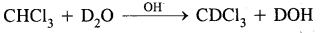Question 39.
What is hydrolith? How is it prepared?
Hydrolith is a calcium hydride (CaH2). It can be prepared by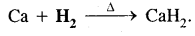Question 40.
Write the structures of two complex metal hydrides which are used as reducing agent in organic synthesis.
Lithium aluminium hydride (LiAlH4) and sodium borohydride (NaBH4).

Question 41.
What type of elements form interstitial hydrides.
d and f-block elements.

Question 42.
Explain why beryllium forms a covalent hydride while calcium forms an ionic hydride?
Because of higher electronegativity (= 1.5). Be forms covalent hydride while due to lower electronegativity (= 1.0), calcium forms ionic hydride.

Question 43.
Write two uses of interstitial hydrides.

1. For storing Hg and
2. Catalysts of hydrogenation reactions.

Question 44.
Explain why electrolysis of ordinary water occurs faster than heavy water.
Due to lower bond dissociation energy of H-O-H bonds in water than D-O-D bonds in D2O, electrolysis of H2O is much faster than of D2O.

Question 45.
Can marine species live in distilled water?
No, because distilled H2O does not contain dissolved oxygen.

Question 46.
Can distilled water be called deionised water?
Yes. Distilled water does not contain any cations and anions.

Question 47.
Which isotope of hydrogen is used as a tracer in organic reactions?
Out of the three isotopes of hydrogen H, D, T both D and T can be used. But due to the radioactive nature of T, it is the only D that is used as a tracer in understanding the mechanism of organic reactions.

Question 48.
Which salts present in water make it permanent hard?
Calcium and magnesium chlorides and sulphates.

Question 49.
Name a process that can remove both temporary and permanent hardness of the water.
Pemiutit process.

Question 50.
Complete the reaction:
Fe(s) + H2O(g) →?
3Fe(s) + 4H2O(g) → Fe3O4(s) + 4H2(g)

### Hydrogen Important Extra Questions Short Answer Type

Question 1.
Hydrogen forms three types of bonds in its compounds. Describe each type of bonding using suitable examples.
Hydrogen forms compounds in three different ways:
1. By loss of electrons as in the reactions of H2 with CuO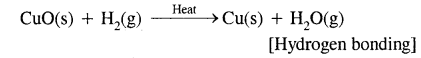2. By gain of electrons as in reactions of H2 with metals.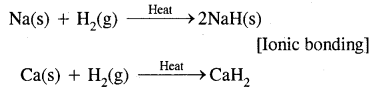3. By sharing of electrons as in the reactions of H2 with halogens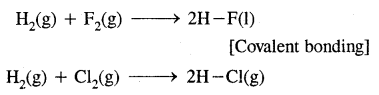Question 2.
Name one example of a reaction in which dihydrogen acts as
(i) an oxidizing agent
As an oxidizing agent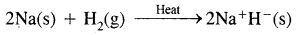Here Na has been oxidized to Na while dihydrogen has been reduced to H+ ion.

(ii) a reducing agent.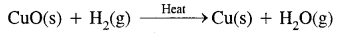Here CuO has been reduced to copper and H2 has been oxidized to H2O.

Question 3.
The process $$\frac{1}{2}$$ H2(g) + e → H (g) is endothermic (DH = +151 kJ mol-1), yet salt- like hydrides are known. How do you account for this?
This is due to the reason that high lattice energy released (energy released during the formation of solid metal hydride from their corresponding gaseous ions, i.e., M+ and H+) more than compensates the energy, needed for the formation of H ions from H2 gas.

Question 4.
Find the volume strength of 1.6 N H2O2 solution.
Strength = Normality × EQuestion wt.
Eq. wt.of H2O2 = 17
∴ Strength of 1.6N H2O2 solution = 1.6 × 17g L-1

Now 68g of H2O2 gives 22400 mL O2 at NTP/STP
∴ 1.6 × 17g of H2O2 will give = $$\frac{22400}{68}$$ × 1.6 × 17
= 8960 mL of O2 at STP
But 1.6 × 17g of H2O2 are present in 1000 mL of H2O2 solution

Hence 1000 mL of H2O2 solution gives 8960 mL of O2 at STP 1 mL of H2O2 will give = 8.96 mL of O2 at STP.

Hence the volume strength of 1.6N H2O2 solution is = 8.96 volume

Question 5.
A sample of hard water is allowed to pass through an anion exchanger. Will it produce lather with soap easily?
No. Ca2+ and Mg2+ ions are still present and these will react with soap to form curdy white ppt. Therefore it will not produce lather with soap solution easily.

Question 6.
Anhydrous Ba02 is not used for preparing H2O2 Why?
BaSO4 formed during the reaction of BaO2 with H2SO4 forms a protective layer around unreacted BaO2 and the reaction stops after some time.

Question 7.
Find the volume strength of the 2N H2O2 solution.
Volume strength = 5.6 × Normality
Volume strength = 5.6 × 2
= 11.2 volumes.

Question 8.
Calculate the concentration in g. L-1 of a 20 volume H2O2 solution.
1 L of 20 volume H2O2 solution on decomposition gives 20L of O2 at STP.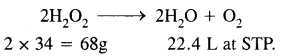Now 22.4 L of O2 at STP is obtained from 68g of H2O2

20L of O2 at STP is obtained from $$\frac{68}{22.4}$$ × 20 = 60.7g

Thus the strength of 20 volume H2O2 solution = 60.7g L-1.

Question 9.
Explain why an oxide ion is called a hard ion?
Oxide ion (O2-) is very small in size and thus cannot be easily polarised and hence it is called a hard oxide ion.

Question 10.
Statues coated with white lead on long exposure to the atmosphere turn black and the original colour can be restored on treatment with H2O2. Why?
On long exposure to the atmosphere, white lead is converted into black PbS due to the action of H2S present in the atmosphere.
PbO2 + 2H2S → PbS + 2H2O

On the treatment of such blackened statues with H2O2, the black PbS gets oxidised to PbSO4 and the colour is restored.
PbS + 4H2O2 → PbSO4 + 4H2O.

Question 11.
A mixture of hydrazine and H2O2 with Cu(II) catalyst is used as a rocket propellant. Why?
The reaction between hydrazine (N2H4) and H2O2 is highly exothermic and is accompanied by a large increase in the volumes of the products and hence the mixture is used as a rocket propellant.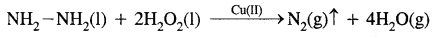Question 12.
Calculate the volume of 10 volume H2O2 solution that will react with 200 ml. of 2N KMnO44 in an acidic medium.
Normality 10 volume H2O2 = $$\frac{10 \times 68}{22.4 \times 17}=\frac{10}{5.6}$$N J
Applying normality equation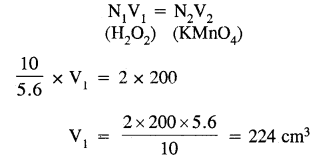Question 13.
What is water gas? How is it prepared?
An equimolar mixture of CO and H is called water gas. It is prepared by passing steam over a red hot iron.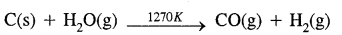Question 14.
The boiling point of H2O is higher than that of H2S. Explain.
Due to intermolecular H-bonding in H2O2 extra energy in the form of heat is required to break these H-bonds due to which H2O boils at a higher temperature than H2S which does not have H-bonding.

Question 15.
Write two uses of interstitial hydrides.
Two important uses to which interstitial hydrides are put are:

1. storing H2 gas
2. Catalysts for hydrogenation reactions.

Question 16.
What is meant by auto protolysis of water?
Auto-protolysis of water means that two molecules of water react with each other through proton transfer, i.e. one acts as the acid and the other acts as a base. The molecule which accepts a proton is converted into H3O+ while the other which loses a proton is converted into OH ion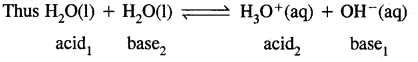Question 17.
What is the difference between hydrolysis and hydration?
Interaction of H+ ions and OH ions of H2O with anions and cations of the salt respectively to give an acidic or a basic solution is called hydrolysis. For example: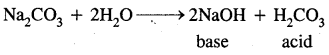Hydration,.on the other hand, means the addition of water to ions or molecules to form hydrated ions or hydrated salts. For examples:Question 18.
Complete the following equations:
(i) Fe(s) + H2O(g) →?
3Fe(s) + 4H2O(g) → Fe3O4(s) + 4H2(g)

(ii) PbS(s) + H2O2(aq)→?
PbS(s) + 4H2O2(aq) → PbSO4(s) + 4H2O

(iii) MnO4(aq) + H2O(g) →?
MnO4-(aq) + 5H2O2(aq) + 6H+ → 2MnO+2+ 8H2O + 5O2

Question 19.
Discuss the importance of heavy water in a nuclear reactor.
Heavy water is used as a moderator in nuclear reactors because it slows down the fast-moving neutrons & therefore helps in controlling the process of nuclear fission. It has also been used as a tracer compound to study the mechanism of many chemical reactions.

Question 20.
Distinguish clearly between:
(a) Hard & soft water.

 S.N. Hard water Soft water 1. It does not give lather with soap freely. 1. It readily forms lather with soap. 2. Magnesium & calcium salts are present in it. 2. It is free from magnesium & calcium salts.

(b) Temporary hardness & permanent hardness of the water.

 S.No. Temporary hardness Permanent hardness 1. This is due to calcium & magnesium bicarbonates in the water. 1. This is due to calcium & magnesium chlorides & sulphates. 2. It can be removed by boiling water. 2. It cannot be removed by boiling water.

Question 21.
Explain the correct context in which the following terms are used:
(a) diprotium
Diprotium: It is used for the correct term for Hr

(b) dihydrogen
Dihydrogen: The term dihydrogen is used for the H2 molecule while referring to the isotopic mixture with natural abundance for H & D.

(c) proton
Proton: It is used for H+.

(d) hydrogen
Hydrogen: It is used in relation to the isotope.

Question 22.
Hydrogen forms three types of bonds in its compound. Describe each type of bonding using suitable examples.
(a) Ionic bond: The binary hydrides of alkali metals like (LiH, KH & NaH otc.) form an ionic bond. They on electrolysis give hydride ion (H)
NaH(s) + H2O(aq) → H2(g) + NaOH(aq)
Na+ + H → NaH

(b) Metallic bond: d & f block elements (Metals) form metallic bonds.
Example: SeH2, YH2 & LaH3 etc.

(c) Covalent bond: With p-block elements hydrogen form a covalent bond. For example,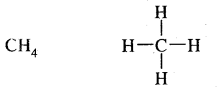Question 23.
Why do lakes freeze from the top to the bottom?
There are intermolecular hydrogen bonding in H2O molecule. The density of water is greater than ice. It may be noted that at 4°C water has maximum density. In the severe cold, the upper layer of the seawater freezes & the heavier water (density more than that of ice) is present below the surface of the ice. Due to this sea animals can live safely in the water.

Question 24.
Why does elemental hydrogen react with other substances only slowly at room temperature?
The high bond energy of di-hydrogen (436 kJ mol-1) makes it a very stable molecule & therefore its reaction with other elements are slow at room temperature.

Question 25.
Why is water an excellent solvent for ionic or polar substances?
Water molecules are highly polar. When water molecules interact with ions of an ionic compound, a large amount of hydration energy is released. The hydration energy is more than the energy needed to overcome the interionic attractions of the ionic compounds as well as the energy needed to break the hydrogen-bonded, association of water molecules. Therefore, the ionic compounds dissolve readily in water.

When water molecule interacts with strongly polar substances, the hydration energy released is sufficient to break the molecules of polar substances into ions as well as to break the hydrogen-bonded, association structure of water molecules. Therefore, water is an excellent solvent for polar compounds.

Question 26.
How is hydrogen obtained from:
(i) Nitric acid
By action of Mg on cold water & dill. HNO3
Mg + 2HNO3(aq) → Mg(NO3)2 + H2

(ii) Alcohol
By action of Na or K on alcohol
2C2H5OH + 2Na → 2C2H5ONa + H2

(iii) Ammonia?
Write a chemical equation in each case.
By passing NH3 over heated Na or K 2Na + 2NH3 → 2NaNH2 + H2

Question 27.
Explain how is dil. solution of hydrogen peroxide concentrated?
Hydrogen peroxide is concentrated by its distillation under reduced pressure at a temperature below 333K & the absence of heat metal impurities. 90% H2O2 is obtained by this method. For further concentration, the solution is cooled to about 263K when crystals enriched in hydrogen peroxide separate out. This process of fractional crystallisation is repeated to get 100% H2O2

Question 28.
Describe how is strength of hydrogen peroxide expressed?
Strength of H2O2 as a percentage: H2O2 in solution (W/V), 70%. H2O2 means 70g of H2O2 is present in 100 g of solution.

Volume strength: Strength of hydrogen peroxide is expressed as the volume of oxygen liberated at S.T.P. given by ml. of a sample of hydrogen peroxide on decomposition into water & oxygen, for example, 30 volumes H2O2 means that 30 ml O2 is obtained at S.T.P. by decomposing 1 ml of H2O22 solution.

Question 29.
What happens when:
(i) Barium peroxide is treated with cold dilute H2SO4.
H2O2 is obtained:
BaO2. 8H2O + H2SO4 → BaSO4 ↑ + H2O2 + 8H2O

(ii) Sodium peroxide is treated with cold dilute H2SO4 & the resulting mixture is cooled below 273 K.
H2O2 is obtained: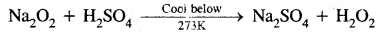(iii) Barium peroxide is treated with Phosphoric acid.
H2O2 is obtained:
3BaO2 + 2H3PO4 → Ba3(PO4)2 + 3H2O2

Question 30.
Why is water an excellent solvent for ionic or polar substances?
Water is a polar solvent with a high dielectric constant. Due to the high dielectric constant of water, the coelomic force of attraction between cations & anions gets weakened. Thus, a water molecule is able to remove ions from the lattice site using ion dipole forces easily. The dissolution of ionic/polar substances in water is further favoured by the hydrations of ions by the water molecule.

### Hydrogen Important Extra Questions Long Answer Type

Question 1.
(a) Compare atomic hydrogen with nascent hydrogen.
Comparison of atomic and nascent hydrogen

The main point of differences are:

1. Nascent hydrogen can be produced even at room temperature but atomic hydrogen is produced only at very high temperature.
2. Nascent hydrogen can never be isolated, but atomic hydrogen can be isolated.
3. The reducing power of atomic hydrogen is much greater than that of nascent hydrogen.

In general reactivity of the three forms of hydrogen increases in order.
Molecular hydrogen (H2) < Nascent hydrogen < Atomic hydrogen.

(b) What is (i) active hydrogen
Active Hydrogen: It is obtained by subjecting a stream of molecular hydrogen at ordinary temperature to silent electric discharge at about 30,000 volts. It is very reactive in nature (half-life = 0.33 second, and combines directly at ordinary temperatures with Pb and S forming their hydrides

(ii) heavy hydrogen? How are they formed?
Heavy hydrogen: It is manufactured by the electrolysis of heavy water containing a little of IT SO, or NaOH to make the solution conducting.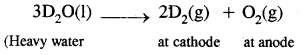In the laboratory, it can be prepared by the action of heavy water on sodium metal.
2D2O(l) + 2Na(s) → 2NaOD(aq) + D2(g).

Question 2.
How is the solution of H2O2 concentrated?
The concentration of hydrogen peroxide: Hydrogen peroxide obtained by any method is always in the form of a dilute solution. Great care is to be taken for concentrating its solution because it is unstable and decomposes on heating.
2H2O2 → 2H2O + O2

The decomposition of H2O2 is catalysed by the ions of heavy metals present as impurities.

The solution of H2O2 is concentrated by the following methods.
1. By careful evaporation on a water bath: A dilute solution of H2O2 is taken in a shallow evaporating dish and is heated at 313K – 323 K. Water evaporates slowly and a hydrogen-peroxide solution of about 15 – 50% strength is obtained.

2. By dehydration in a vacuum desiccator: The dilute (50 %) solution of H2O2 obtained as above, is further concentrated by placing the same in a vacuum desiccator containing concentrated H2SO4 as a dehydrating agent. Here, water vapours are absorbed by concentrated sulphuric acid. This is shown in the diagram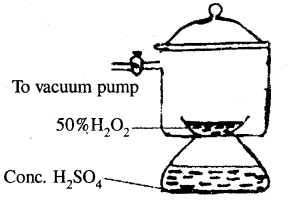(Concentration of H2O2 in vacuum desiccator)

3. By distillation under reduced pressure: The solution of hydrogen peroxide is further concentrated by subjecting it to distillation under reduced pressure. The solution is distilled at 308 – 313 K under a reduced pressure of 15 mm Hg. Water present in the solution distils over leaving behind about 98 – 99% concentrated solution of hydrogen peroxide.

4. By crystallisation: The last traces of water present in H2O are removed by freezing it in a freezing mixture of solid CO2 and others. The crystals of hydrogen peroxide separate out. These crystals are removed, dried and then remitted to obtain 100% pure hydrogen peroxide.

5. Storage of hydrogen peroxide: In order to check the decomposition of hydrogen peroxide, a small amount of acetanilide (i.e. negative catalyst) is added to it before storing the hydrogen peroxide.

Hydrogen peroxide cannot be concentrated by distillation at ordinary pressure because it undergoes decomposition into water and oxygen as it is a highly unstable liquid. It decomposes even on long-standing or on heating.

Question 3.
What are the different methods used for the softening of hard water? Explain the principle of each method.
Hard water can be softened by the following methods depending upon the nature of hardness.
(a) Temporary hardness:
1. By boiling: It can be removed by merely boiling the water. Boiling decomposes the bicarbonates to give carbon dioxide and insoluble carbonates, which can be removed by filtration.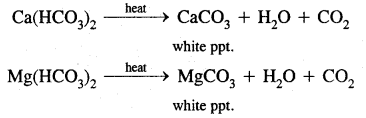2. Clark’s process: Temporary hardness can be removed by the addition of a calculated amount of lime, whereupon magnesium and/or calcium carbonates is precipitated.
Ca(HCO3)2 + Ca(OH)2 → 2CaCO3 + 2H2O
Mg(HCO3)2 + Ca(OH)2 → CaCO33 + MgCO3 + 2H2O

(b) Permanent hardness:
1. With sodium carbonate: On treatment with washing soda, Ca2+ and Mg2+ in hard water are precipitated. The precipitate of the insoluble carbonates thus formed is removed by filtration.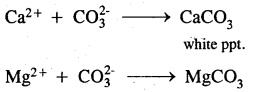2. Ion-exchange method: The common substance used for this process is zeolite which is hydrated sodium aluminium silicate, NaAl(SiO)2, The exchange occurs when passing over the zeolite bed, sodium ions from zeolite are replaced by calcium and magnesium ions. Thus
Na(Ze) + Mg2+ → (Ze)2Ca + 2Na+
2NaZe + Mg2+ → (Ze)2Mg + 2Na+

when all the sodium ions of the zeolite have been replaced, the zeolite is said to be exhausted. It can be regenerated by treatment with a strong solution of sodium chloride.
2Na + (Ze)2Ca → 2ZeNa + Ca2+.

Question 4.
Show how hydrogen peroxide can function both as an oxidising and a reducing agent.
Oxidising properties: H2O2 has a tendency to accept electrons in chemical reactions and thus behaves as an oxidising agent in both acidic and alkaline medium.
H2O2 → H2O + O
In acidic medium
H2O2 + 2H+ + 2e → 2H2O

In alkaline medium
H2O2 + OH + 2e → 3OH
Example:
(a) In acidic medium:
2Fe2+ + 2H+ + H2O2 → 2Fe3+ + 2H2O
(b) In alkaline medium:
3Cr3+ + 4H2O2 + 100H → 3CrO42- + 8H2O

Reducing properties: H2O2 can give electrons in a few reactions and thus behaves as a reducing agent.
In acidic medium
H2O2 → O2 + 2H+ + 2e

In alkaline medium
H2O2 + 2OH → 2H2O + O2 + 2e

Reducing property in acidic medium:
2MnO42- + 6H+ + 5H2O2 → 2Mn2+ + 8H2O + 5O2

Reducing property in basic medium:
2Fe3+ + H2O2 + 2OH → 2Fe2+ + O2 + 2H2O

### Hydrogen Important Extra Questions Numerical Problems

Question 1.
Calculate the percentage strength & strength in g/L of 10 volume hydrogen peroxide solution.
H2O2 decomposes on heating according to the equation:From the equation
22.4L of O2 at N.T.P are obtained from 68g of H202

∴ 10 ml of O2 at N.T.P will be obtained from $$\frac{68}{22400}$$ × 10g of H2O2
But 10 ml of O2 at N.T.P are produced from 1 ml. of 10 volume H2O2 solution.

Thus 1 ml of 10 volume H2O2 solution contains $$\frac{68}{22400}$$ × 10 g of H2O2
∴ 100 ml. of 10 volume H2O2 solution will contain
$$\frac{68}{22400}$$ × $$\frac{10}{1}$$ × 100 = 3.036g .

Thus a 10 volume H2O2 solution is approx. 3%
Alternatively, 1000 ml of 10 volume of H2O2 will contain H2O2
$$\frac{68}{22400}$$ × 10 × 1000 = 30.36g

Therefore, strength of H2O2 in 10 volume H2O2 is 30.36 g/L

Question 2.
Calculate the normality of 20 volume hydrogen peroxide solution.
Step-I: To calculate the strength in g/L of 20 volume H2O2 solution.
By definition, 1L of 20 volume H2O2 solution on decomposition gives 20 litres of oxygen at N.T.P. consider the chemical equation.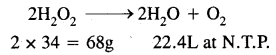Now 22.4 litres of 02 at N.T.P. will be obtained from H2O2
$$\frac{68}{22400}$$ × 20g = 60.7g

Thus, the strength of 20 volume H2O2 solution is 60.7 g/l

Step-II: To calculate the equivalent wt. of H2O2 consider the chemical equation,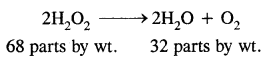From the above equation, 32 parts by wt. of oxygen are obtained from 68 parts by wt. of H2O2
∴ 8 parts by wt. of oxygen will be obtained from
$$\frac{68}{32}$$ × 8 = 17 parts by wt. of H2O2
∴ Equivalent wt. of H2O2 = 17

Step-III: To calculate the normality = $$\frac{\text { Strength }}{\text { Eq. wt. }}=\frac{60.7}{17}$$ = 3.57
Hence normality of 20 volume H2O2 solution = 3.57N

Question 3.
Find the volume strength of the 1.6N H2O2 solution.
We know that strength = Normality × Eq. wt. of H2O2

∴ Strength of 1.6N H2O2 solution = 1.6N × 17
Now 68g of H2O2 gives 22400 ml O2 at N.T.P.

∴ 1.6 × 17g of H2O2 will give
$$\frac{22400}{68}$$ × 1.6 × 17 = 8960 ml of O2 at N.T.P.

But 1.67 × 17g of H2O2 are present in 1000 ml of H2O2 solution.
Hence 1000 ml of H2O2 solution gives 8960 ml of O2 at N.T.P.

∴ 1 ml of H2O2 solution will give = $$\frac{8960}{1000}$$
= 8.96 ml of O2 at N.T.P.
Hence the volume strength of 1.6N H2O2 solution = 8.96 volume.

Question 4.
Calculate the amount of H2O2 present in 10 ml of 25 volume H2O2 solution.
ml. of 25 volume H2O2 liberate O2
10 × 25 = 250 ml. at N.T.P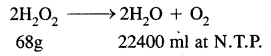∴ Amount of H2O2 that will liberate 250 ml of O2 at N.T.P.
= $$\frac{68 \times 250}{22400}$$ = 0.759 g

Question 5.
10 ml of a given solution of H2O2 contains 0.91 g of H2O2 Express its strength in volume.
68g of H2O2 produce O2 = 22400 ml at N.T.P.

∴ 0.91g of H2O2 will produce O2
$$\frac{22400 \times 0.91}{68}$$ = 300 ml at N.T.P.

Volume strength = $$\frac{300}{10}$$ = 30

Question 6.
Calculate the strength in volumes of a solution containing 30.36 g/litre of H2O2.30.36g of K2O2 will produce $$\frac{22.4}{68}$$ × 30.46